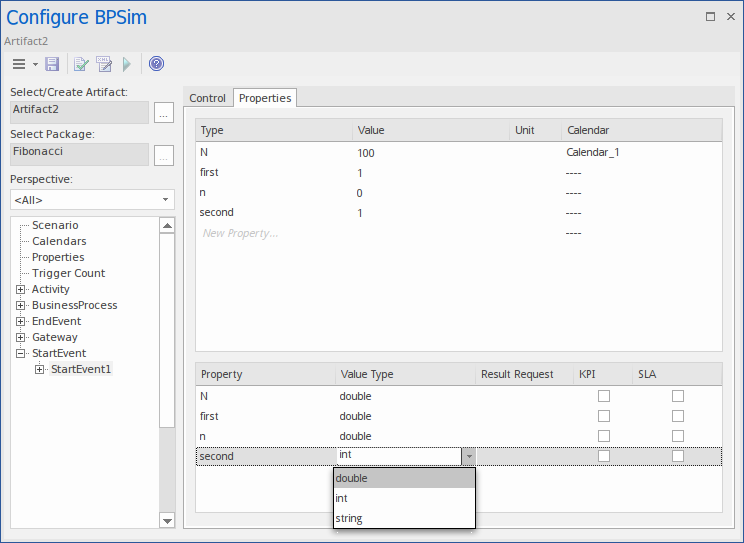#### Please note : This help page is not for the latest version of Enterprise Architect. The latest help can be found here.

 Prev Next

# BPSim Execution Engine - Simulation Language

The BPSim Execution Engine supports simulation on XPath 1.0 or Java, where the appropriate language is defined as the Expression Language in the simulation configuration. It also supports the use of process instance data in BPSim Property Parameters, where the actual value is only determined during execution.

## XPath 1.0 Operators

These operators can be used in BPSim expression parameters.

Operator

Description

|

The Union operator, used for resource acquisition.

Example: getResource('w1',1) | getResource('w2',1)

+

Example: 4 + 6

-

Subtraction.

Example: 6 - 4

*

Multiplication.

Example: 6 * 4

div

Division.

Example: 8 div 4

=

Equality.

Example: 4 = 4 (True)

!=

Not Equal.

Example: 5 != 3

<

Less than.

Example: 6 < 9

<=

Less than or equal to.

Example: x <= 6

>

Greater than.

Example: 9 > 6

>=

Greater than or equal to.

Example: n >= 7

or

Alternative.

Example: n = 6 or n <= 6

and

Combination.

Example: n = 5 and m < 8

mod

Modulus division.

Example: 5 mod 2

getProperty

Get a property value.

Example: getProperty ("amount")

getResource

Get a resource assignment.

Example: getResource ('w1',1)

## Note

The Expression Language can be set in the Configure BPSim window, on the 'Configure' tab; the two options 'XPath 1.0' and 'Java' are available as values of the 'Expression' parameter.

If you select 'Java', you must set the property 'JDK Home' to a valid JDK directory.

Tip: You can use {PropertyParameterName} as a short form of getProperty('PropertyParameterName'), which is useful when writing the value for the expressions; for example:

{n} < {N}     instead of      getProperty('n') < getProperty('N')

The short form of the getProperty operator can be used in both XPath 1.0 and Java.

## BPSim Property Parameters

From Enterprise Architect release 13.0 onwards, BPSim property parameters can hold process instance data to which no value is assigned until run time. You can define the property parameter type on the 'Properties' page of the Configure BPSim window; the supported types are 'int', 'double' and 'string'.